# Symmetric matrix

This article defines a property that can be evaluated for a square matrix. In other words, given a square matrix (a matrix with an equal number of rows and columns) the matrix either satisfies or does not satisfy the property.
View other properties of square matrices

## Definition

### Verbal definition

A symmetric matrix is a square matrix that equals its own matrix transpose. Explicitly, a square matrixis termed a symmetric matrix if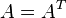.

### Algebraic definition

Supposeis a positive integer and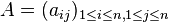is amatrix. We say thatis symmetric if the following holds:Note that thecondition is not required; the above definition is equivalent to: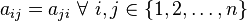Finally, because the equality condition is symmetric in, it suffices to check it for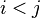, so the above definition if equivalent to:## Encoding

We can encode a symmetric matrix by simply storing the entries on or above the diagonal. This is a total ofentries instead of the total of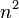entries.

## Small cases

For the equal entries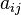and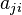,, we will useas the label. This is purely a matter of convention.General description ofsymmetric matrix Number of free parameters (equals)
11
2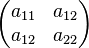3
3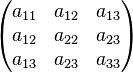6

## Matrix operations

### Invariant computation

For both the trace and the determinant, there does not seem to be a simpler way of computing them than the standard approach for square matrices.

### Operations

Matrix operation Description in terms of encoding Number of additions Number of multiplications Number of inverse computations Set of symmetric matrices closed under operation?
Addition of two symmetric matrices Add the corresponding entries of the two matrices0 0 Yes
Multiplication of two symmetric matrices Usual matrix multiplication algorithms; symmetry does not seem to offer speedup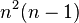for naive matrix multiplicationfor naive matrix multiplication 0 No

## Relation with other properties

### Stronger properties

Property Meaning Proof of implication Proof of strictness (reverse implication failure) Intermediate notions
scalar matrix scalar multiple of identity matrix Bisymmetric matrix, Diagonal matrix|FULL LIST, MORE INFO
diagonal matrix all off-diagonal entries are zero |
bisymmetric matrix both symmetric and centrosymmetric |